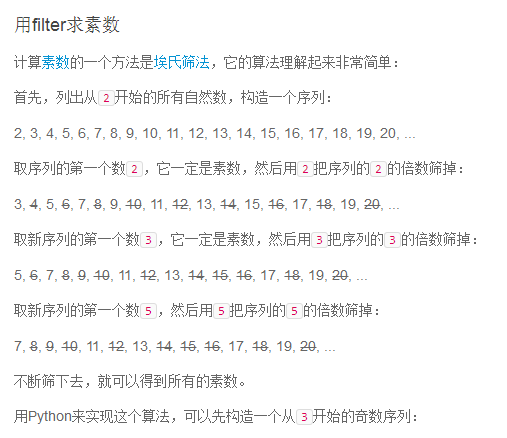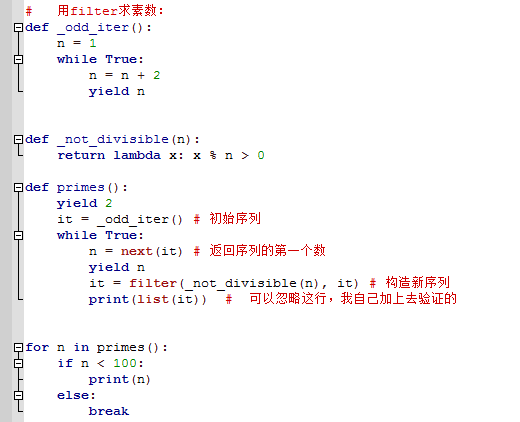2018-07-26 02:23

# 用filter求素数：• 写回答
• 好问题 提建议
• 关注问题
• 收藏
• 邀请回答

#### 3条回答默认 最新

•threenewbee 2018-07-26 03:13
已采纳

你修改了题目？
根据你题目的算法，用筛选法也可以

`````` def _odd_iter():
n = 1
while True:
n = n + 2
yield n

def _not_divisible(n):
return lambda x: x % n > 0

def primes():
yield 2
it = _odd_iter()
while True:
n = next(it)
yield n
it = filter(_not_divisible, it)

for n in primes():
if n < 100:
print(n)
else:
break;
``````

之所以不会无限执行，是因为这是生成器，所以for n in primes():这个循环其实是

`````` it = primes()
while True:
x = next(it)
print(x)
if (x > 100): break
``````

而每次调用next，

def primes()中的代码会走到下一次yield就暂停了。

已采纳该答案
评论
解决 无用
打赏 举报
•threenewbee 2018-07-26 03:02
``````from itertools import *

def _odd_iter():
n = 1
while True:
n = n + 2
yield n

def divisible(n):
return lambda x: x % n == 0

def primes():
yield 2
for x in _odd_iter():
for y in range(3, x + 3):
if x == y:
yield x
if divisible(y)(x):
break;

for n in takewhile(lambda x: x < 100, primes()):
if n < 100:
print(n)
else:
break;
``````
评论
解决 无用
打赏 举报
•threenewbee 2018-07-26 03:03

2
3
5
7
11
13
17
19
23
29
31
37
41
43
47
53
59
61
67
71
73
79
83
89
97

Process finished with exit code 0

评论
解决 无用
打赏 举报